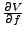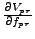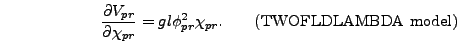Next: Effective Masses Up: Writing Down the Equations Previous: The Program Potential

### The Potential Derivative

The equations of motion for the fields all involve the term. When these equations are derived in program variables in section 6.1 this term simply becomes. So once you have written down the program potential all you have to do is take its derivative. For the TWOFLDLAMBDA model this gives(4.12)(4.13)